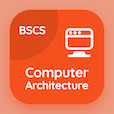Computer Science Online Courses

Digital Logic Design Quizzes

Digital Logic Design Quiz PDF - Complete

# Half Adders Multiple Choice Questions p. 62

Study Half Adders multiple choice questions and answers, half adders quiz answers PDF 62 to study Digital Logic Design course online. Combinational Logics MCQ trivia questions, half adders Multiple Choice Questions (MCQ) for online college degrees. "Half Adders MCQ" PDF eBook: introduction to asynchronous sequential logic, boolean functions implementations, introduction to synchronous sequential logic, logic gates in digital logic design, half adders test prep for online college courses.

"Two-bit addition is done by" MCQ PDF: carry sum adder, ripple carry adder, full adder, and half adder for online computer engineering programs. Learn combinational logics questions and answers to improve problem solving skills for online bachelor's degree computer science.

MCQ: Two-bit addition is done by

MCQ: Which one of the following is not a gate?

AND
OR
NOT
XNOT

MCQ: Sequential circuits are

Synchronous
Asynchronous
signals
both a and b

MCQ: AND gates are converted to NAND gates using

invert OR
AND invert
NAND invert
NOR invert

MCQ: Asynchronous sequential logic circuit not uses

inputs
outputs
clock pulses
time

### More Quizzes from Digital Logic Design Course